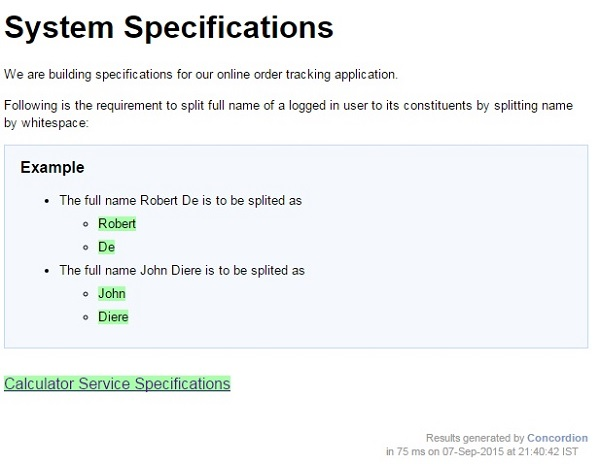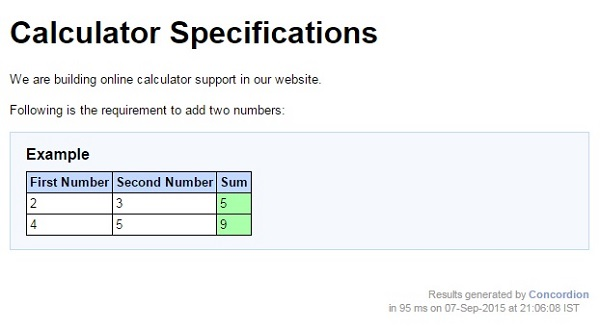# Concordion - Run Command

Concordion run command can be used to link multiple specifications together and display them at one central page. This command can run all the specifications, while displaying the link's background in green / red / gray as appropriate.
Now we are going to create two specifications and link them together. We'll be reusing the specifications created in Concordion - Execute on List and Concordion - Execute on Table chapters as System Specifications and Calculator Specifications.

### Example

Let us have a working Eclipse IDE in place and follow the steps given below to create a Concordion application −
StepDescription
1Create a project with a name concordion and create a package com.tutorialspoint under the src folder in the created project.
2Add the required Concordion libraries using Add External JARs option as explained in the Concordion - First Application chapter.
3Create Java class System under the com.tutorialspoint package.
4Create Fixture classes SystemFixtureCalculatorFixture under the specs.tutorialspoint package.
5Create Specification html files System.htmlCalculator.html under the specs.tutorialspoint package.
6The final step is to create the content of all the Java files and specification file and run the application as explained below.
Here is the content of System.java file −
```package com.tutorialspoint;
import org.concordion.api.MultiValueResult;

public class System {
MultiValueResult result = new MultiValueResult();
result.with("firstName", words).with("lastName", words);
return result;
}

public int sum(int firstNumber, int secondNumber) {
return firstNumber + secondNumber;
}
}```
Following is the content of SystemFixture.java file −
```package specs.tutorialspoint;

import org.concordion.api.MultiValueResult;
import org.concordion.integration.junit4.ConcordionRunner;
import org.junit.runner.RunWith;
import com.tutorialspoint.System;

@RunWith(ConcordionRunner.class)

public class SystemFixture {
System system = new System();
}
}```
Following is the content of CalculatorFixture.java file −
```package specs.tutorialspoint;

import org.concordion.integration.junit4.ConcordionRunner;
import org.junit.runner.RunWith;
import com.tutorialspoint.System;

@RunWith(ConcordionRunner.class)

public class CalculatorFixture {
System system = new System();
public int sum(int firstNumber, int secondNumber) {
return system.sum(firstNumber, secondNumber);
}
}```
Following is the content of System.html file −
```<html xmlns:concordion = "http://www.concordion.org/2007/concordion">
<link href = "../concordion.css" rel = "stylesheet" type = "text/css" />

<body>
<h1>System Specifications</h1>
<p>We are building specifications for our online
order tracking application.</p>
<p>Following is the requirement to split full name of a
logged in user to its constituents by splitting name by whitespace:</p>

<div class = "example">
<h3>Example</h3>

<ul>
<li>The full name <span concordion:execute = "#result = split(#TEXT)">
Robert De</span> is to be splited as
<ul>
<li><span concordion:assertEquals = "#result.firstName">
Robert</span></li>
<li><span concordion:assertEquals = "#result.lastName">
De</span></li>
</ul>
</li>

<li>The full name <span concordion:execute = "#result = split(#TEXT)">
John Diere</span> is to be splited as
<ul>
<li><span concordion:assertEquals = "#result.firstName">
John</span></li>
<li><span concordion:assertEquals = "#result.lastName">
Diere</span></li>
</ul>
</li>

</ul>
</div>

<a concordion:run = "concordion" href = "Calculator.html">
Calculator Service Specifications</a>
</body>

</html>```
Following is the content of Calculator.html file −
```<html xmlns:concordion = "http://www.concordion.org/2007/concordion">
<link href = "../concordion.css" rel = "stylesheet" type = "text/css" />

<body>
<h1>Calculator Specifications</h1>
<p>We are building online calculator support in our website.</p>
<p>Following is the requirement to add two numbers:</p>

<div class = "example">
<h3>Example</h3>

<table>
<tr>
<th>First Number</th>
<th>Second Number</th>
<th>Sum</th>
</tr>
<tr concordion:execute = "#result = sum(#firstNumber, #secondNumber)">
<td concordion:set = "#firstNumber">2</td>
<td concordion:set = "#secondNumber">3</td>
<td concordion:assertEquals = "#result">5</td>
</tr>
<tr concordion:execute = "#result = sum(#firstNumber, #secondNumber)">
<td concordion:set = "#firstNumber">4</td>
<td concordion:set = "#secondNumber">5</td>
<td concordion:assertEquals = "#result">9</td>
</tr>
</table>

</div>
</body>

</html>```
Once you are done with creating source and specification files, let us run the application as JUnit Test. If everything is fine with your application, then it will produce the following result −
```C:\DOCUME~1\ADMINI~1\LOCALS~1\Temp\concordion\specs\tutorialspoint\System.html
Successes: 2, Failures: 0